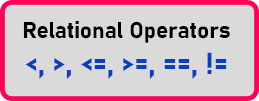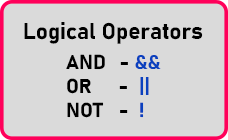# Logical Operators in C++

## Logical Operators in C++ with Examples:

In this article, I am going to discuss Logical Operators in C++ with Examples. Please read our previous article where we discussed Finding Maximum of Two Numbers Program in C++ with Examples.

##### Logical Operators in C++:

Logical operators are useful for writing compound conditional statements. Already in our previous article, we have learned about Conditional Statements. For writing conditional statements we use the following relational operators.We can use these operators for writing the condition as we have written some programs in the previous articles. Now if we have more than one condition then how to write them? Suppose we have two conditions that are ‘x’ is less than ‘y’ as well as ‘x’ is less than ‘z’. Here ‘x’, ‘y’, and ‘z’ are some variables.

So, here we want to combine two conditions. So, if we combine them that will be called a compound conditional statement. The compound conditional statement can be formed by using the following logical operators.NOT is used for negating the statement means if it is true then it will become false or vice versa. AND, OR are used for making a compound conditional statement. In C++, for AND, OR, NOT, symbols are ‘&&’, ‘||’ and ‘!’. Now, let us understand how these logical operators work?

##### Logical AND Operator:

If we use AND how it works. It is logical, so it depends upon its truth values so that we will see using a truth table. Now we have two statements that are ‘I am wearing a t-shirt’, true. And suppose ‘I am wearing cap’ then also true. Then if I say ‘I am wearing a t-shirt and am wearing a cap’. Will this complete statement be true or false? It will be true. So let us look at the truth table. Let us have two variables ‘x’ and ‘y’. We know ‘0’ means false and ‘1’ means true, so the truth table isThis is the truth table for the “AND” logical operator. So, now we can understand that AND will be true if both those statements are true. If any of the statements is false then the result of AND will be false. If the value of both the variables is true then only the result of AND will be true. This logical operation works on the ‘boolean’ value so that is true and false only.

##### Logical OR Operator:

We will use the same example to understand OR operator. The Truth table for Logical OR Operator is as follows.Here you can see that the result of ‘x OR y’ will be true if any of the variable values are true and the result will be false if both the variables are false. So, the difference between ‘AND’ and ‘OR’ is, in ‘AND’ if one is false then everything is false and in ‘OR’ if one is true then everything is true. So, this is how logical operators are useful for combining conditions. Then one last thing we have to show you that is ‘NOT’.

##### Logical NOT Operator:

The Logical ‘NOT’ Operator is used for negating a statement. Negating means if it is true then it will become false or if it is false then it will become true. The Truth table for Logical NOT Operator is as follows.So, if x is true then ‘~x’ will be false, or if x is false then ‘~x’ will be true. So, it looks simple and now it may look useless but it is very useful. So that’s all about these logical operators. These are used for writing compound conditional statement.

##### Logical Operator Precedence and Associativity:

The Logical-Not (!) has more priority than && and ||##### Relation between conditional statements and Logical Operators

As discussed, the logical operators are used when we need to combine two conditions. Hence logical operators have tightly coupled in the case of compound conditional statements.

Example: if(a>10 && b<20) this is the compound conditional statements.

In the next article, I am going to discuss Compound Conditional Statements in C++ with Examples. Here, in this article, I try to explain Logical Operators in C++ with Examples and I hope you enjoy this Logical Operators in C++ with Examples article.